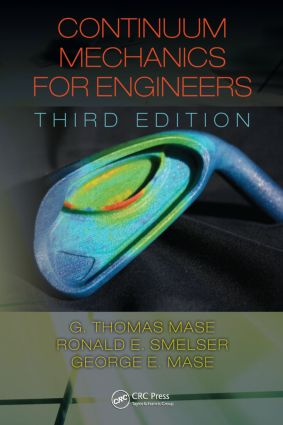Continuum Mechanics for Engineers

3rd Edition

CRC Press

398 pages | 130 B/W Illus.

Purchasing Options:\$ = USD
Hardback: 9781420085389
pub: 2009-07-28
SAVE ~\$28.00
\$140.00
\$112.00
x
eBook (VitalSource) : 9780429139284
pub: 2009-07-28
from \$70.00

FREE Standard Shipping!

Description

Continuum Mechanics for Engineers, Third Edition provides engineering students with a complete, concise, and accessible introduction to advanced engineering mechanics. The impetus for this latest edition was the need to suitably combine the introduction of continuum mechanics, linear and nonlinear elasticity, and viscoelasticity for a graduate-level course sequence. An outgrowth of course notes and problems used to teach these subjects, the third edition of this bestselling text explores the basic concepts behind these topics and demonstrates their application in engineering practice.

Presents Material Consistent with Modern Literature

A new rearranged and expanded chapter on elasticity more completely covers Saint-Venant’s solutions. Subsections on extension, torsion, pure bending and flexure present an excellent foundation for posing and solving basic elasticity problems. The authors’ presentation enables continuum mechanics to be applied to biological materials, in light of their current importance. They have also altered the book’s notation—a common struggle for many students—to better align it with modern continuum mechanics literature. This book addresses students’ need to understand the sophisticated simulation programs that use nonlinear kinematics and various constitutive relationships. It includes an introduction to problem solution using MATLAB®, emphasizing this language’s value in enabling users to stay focused on fundamentals.

This book provides information that is useful in emerging engineering areas, such as micro-mechanics and biomechanics. With an abundance of worked examples and chapter problems, it carefully explains necessary mathematics as required and presents numerous illustrations, giving students and practicing professionals an excellent self-study guide to enhance their skills. Through a mastery of this volume’s contents and additional rigorous finite element training, they will develop the mechanics foundation necessary to skillfully use modern, advanced design tools.

Continuum Theory

Continuum Mechanics

Starting Over

Notation

Essential Mathematics

Scalars, Vectors and Cartesian Tensors

Tensor Algebra in Symbolic Notation - Summation Convention

Indicial Notation

Matrices and Determinants

Transformations of Cartesian Tensors

Principal Values and Principal Directions

Tensor Fields, Tensor Calculus

Integral Theorems of Gauss and Stokes

Stress Principles

Body and Surface Forces, Mass Density

Cauchy Stress Principle

The Stress Tensor

Force and Moment Equilibrium; Stress Tensor Symmetry

Stress Transformation Laws

Principal Stresses; Principal Stress Directions

Maximum and Minimum Stress Values

Mohr’s Circles For Stress

Plane Stress

Deviator and Spherical Stress States

Octahedral Shear Stress

Kinematics of Deformation and Motion

Particles, Configurations, Deformations and Motion

Material and Spatial Coordinates

Langrangian and Eulerian Descriptions

The Displacement Field

The Material Derivative

Deformation Gradients, Finite Strain Tensors

Infinitesimal Deformation Theory

Compatibility Equations

Stretch Ratios

Rotation Tensor, Stretch Tensors

Velocity Gradient, Rate of Deformation, Vorticity

Material Derivative of Line Elements, Areas, Volumes

Fundamental Laws and Equations

Material Derivatives of Line, Surface, and Volume Integrals

Conservation of Mass, Continuity Equation

Linear Momentum Principle, Equations of Motion

Piola-Kirchhoff Stress Tensors, Lagrangian Equations of Motion

Moment of Momentum (Angular Momentum) Principle

Law of Conservation of Energy, The Energy Equation

Entropy and the Clausius-Duhem Equation

The General Balance Law

Restrictions on Elastic Materials by the Second Law of Thermodynamics

Invariance

Restrictions on Constitutive Equations from Invariance

Constitutive Equations

Linear Elasticity

Elasticity, Hooke’s Law, Strain Energy

Hooke’s Law for Isotropic Media, Elastic Constants

Elastic Symmetry; Hooke’s Law for Anisotropic Media

Isotropic Elastostatics and Elastodynamics, Superposition Principle

Saint-Venant Problem

Plane Elasticity

Airy Stress Function

Linear Thermoelasticity

Three-Dimensional Elasticity

Classical Fluids

Viscous Stress Tensor, Stokesian, and Newtonian Fluids

Basic Equations of Viscous Flow, Navier-Stokes Equations

Specialized Fluids

Steady Flow, Irrotational Flow, Potential Flow

The Bernoulli Equation, Kelvin’s Theorem

Nonlinear Elasticity

Molecular Approach to Rubber Elasticity

A Strain Energy Theory for Nonlinear Elasticity

Specific Forms of the Strain Energy

Exact Solution for an Incompressible, Neo-Hookean Material

Linear Viscoelasticity

Viscoelastic Constitutive Equations in Linear Differential Operator Form

One-Dimensional Theory, Mechanical Models

Creep and Relaxation

Superposition Principle, Hereditary Integrals

Harmonic Loadings, Complex Modulus, and Complex Compliance

Three-Dimensional Problems, The Correspondence Principle

Appendices

Index